Скачать презентацию Modelling the convective zone of a utility boiler

1c43c7ca1f26c705669c1e6aac1c127c.ppt

• Количество слайдов: 45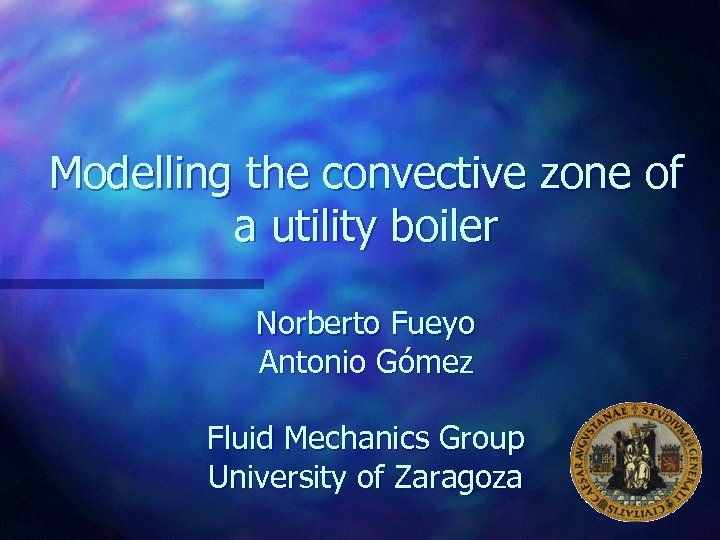Modelling the convective zone of a utility boiler Norberto Fueyo Antonio Gómez Fluid Mechanics Group University of Zaragoza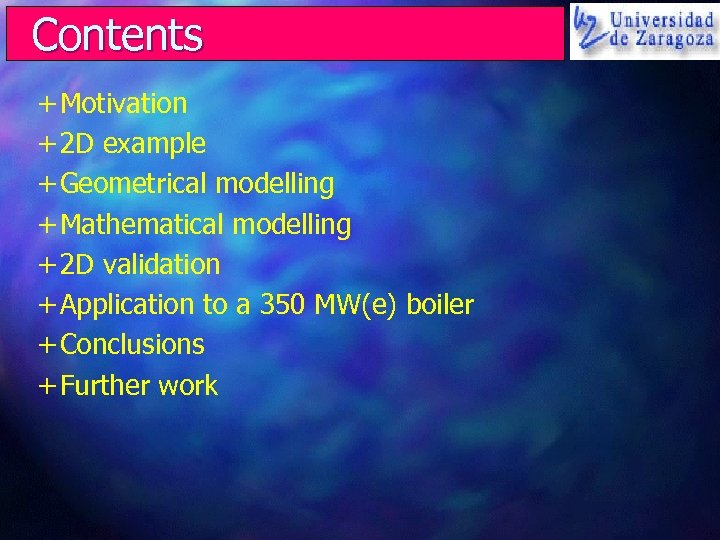Contents + Motivation + 2 D example + Geometrical modelling + Mathematical modelling + 2 D validation + Application to a 350 MW(e) boiler + Conclusions + Further workMotivation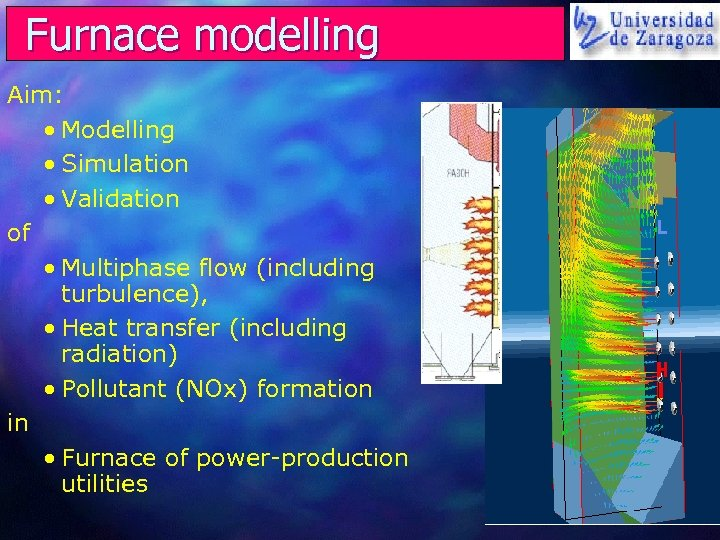Furnace modelling Aim: • Modelling • Simulation • Validation of • Multiphase flow (including turbulence), • Heat transfer (including radiation) • Pollutant (NOx) formation in • Furnace of power-production utilities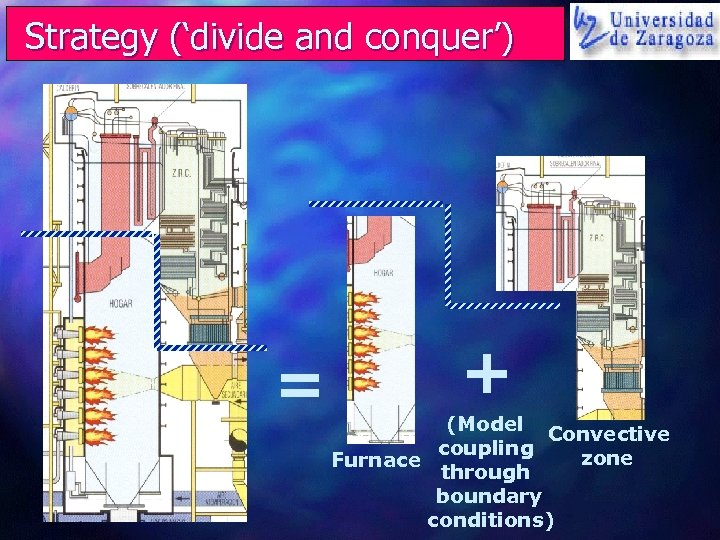Strategy (‘divide and conquer’) = + (Model Convective coupling zone Furnace through boundary conditions)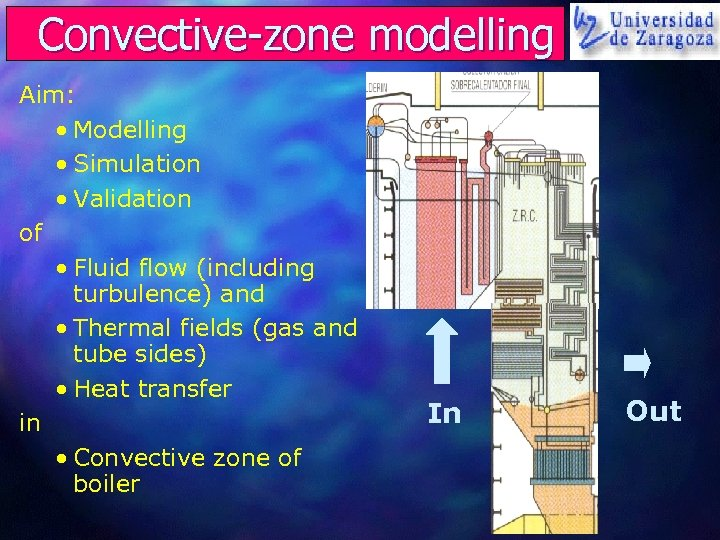Convective-zone modelling Aim: • Modelling • Simulation • Validation of • Fluid flow (including turbulence) and • Thermal fields (gas and tube sides) • Heat transfer in • Convective zone of boiler In Out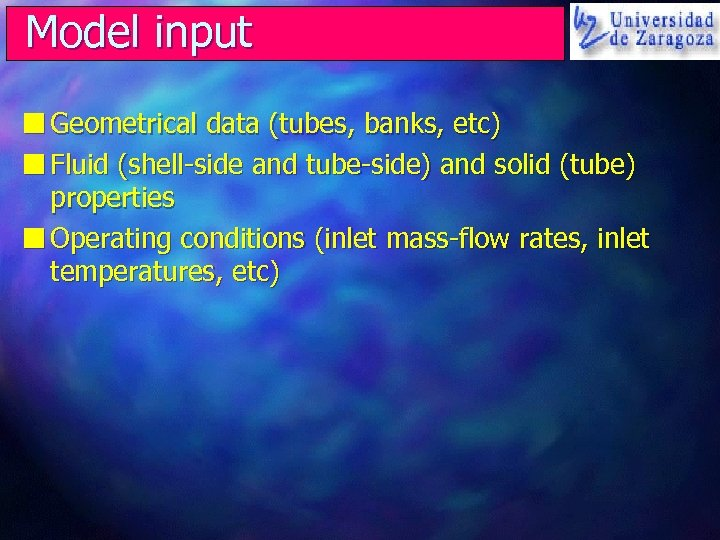Model input n Geometrical data (tubes, banks, etc) n Fluid (shell-side and tube-side) and solid (tube) properties n Operating conditions (inlet mass-flow rates, inlet temperatures, etc)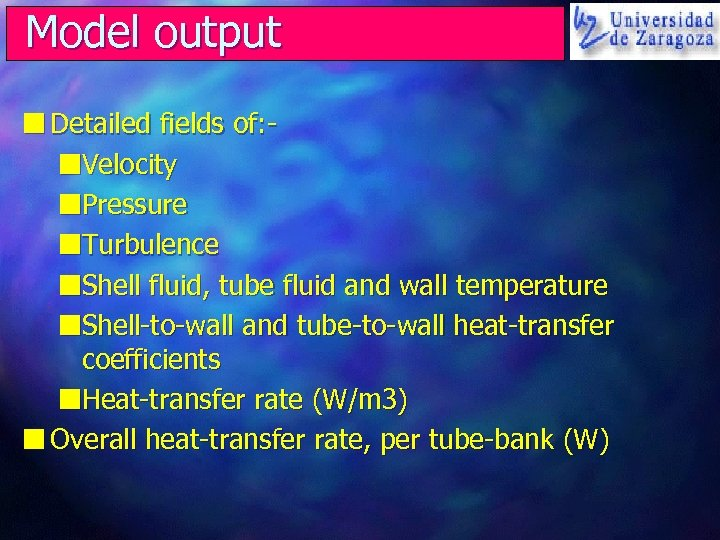Model output n Detailed fields of: n. Velocity n. Pressure n. Turbulence n. Shell fluid, tube fluid and wall temperature n. Shell-to-wall and tube-to-wall heat-transfer coefficients n. Heat-transfer rate (W/m 3) n Overall heat-transfer rate, per tube-bank (W)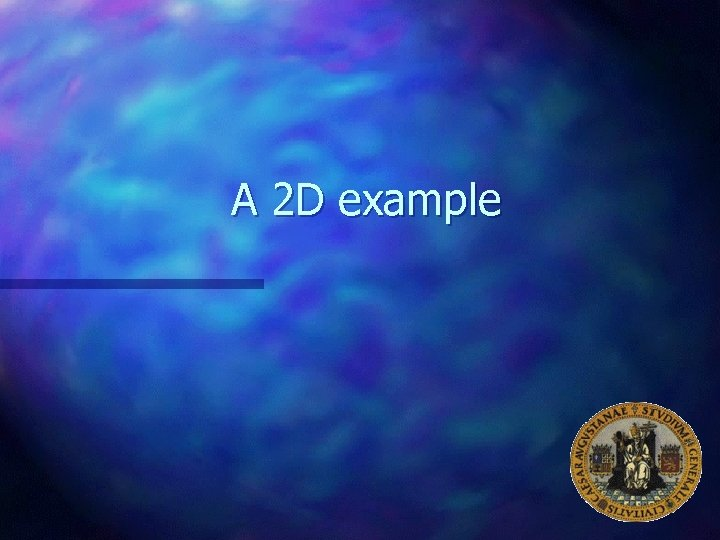A 2 D example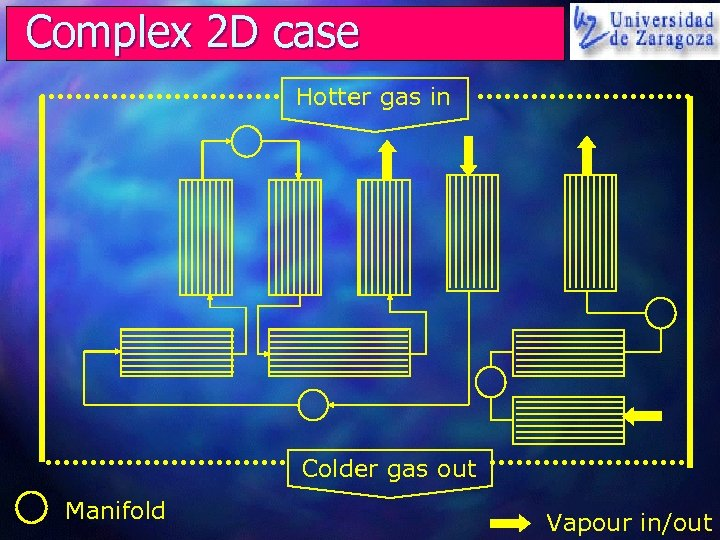Complex 2 D case Hotter gas in Colder gas out Manifold Vapour in/out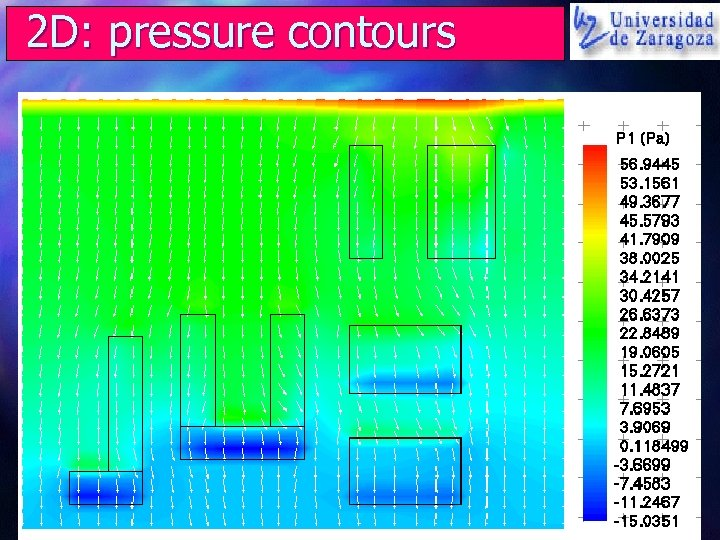2 D: pressure contours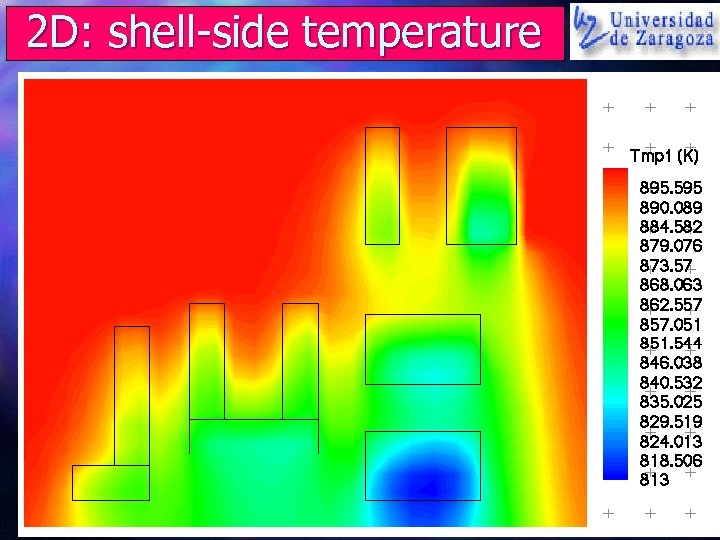2 D: shell-side temperature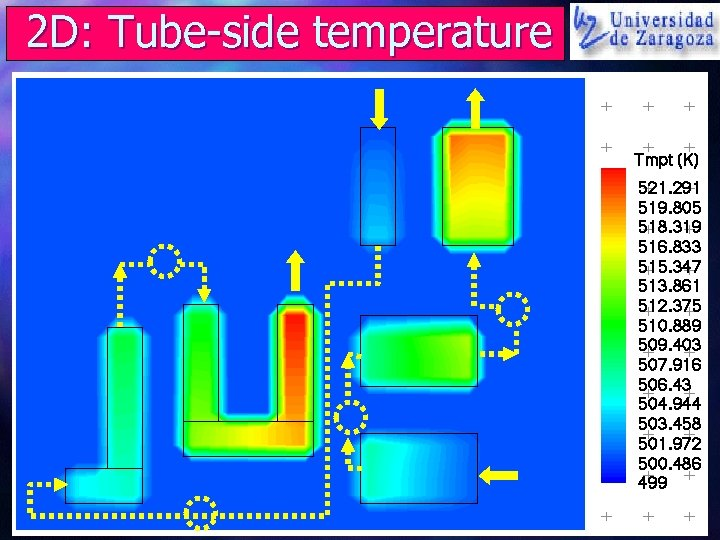2 D: Tube-side temperature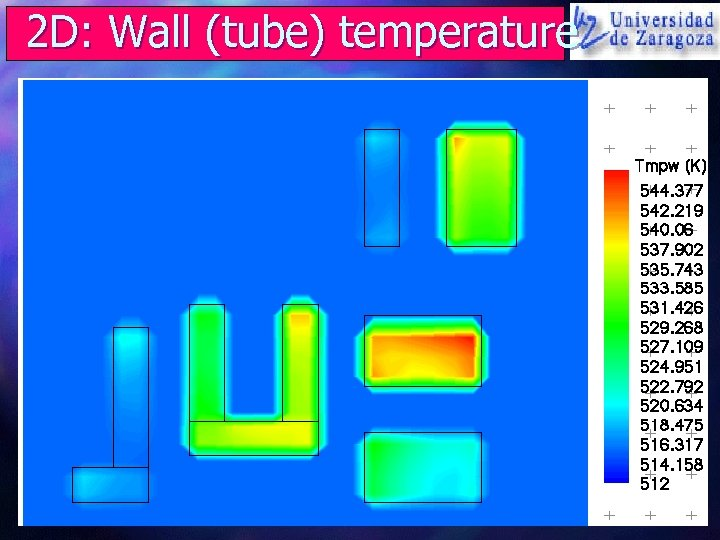2 D: Wall (tube) temperature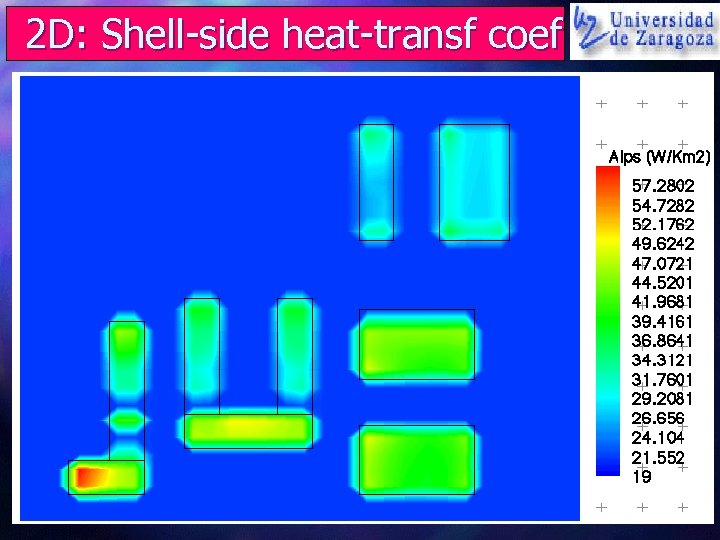2 D: Shell-side heat-transf coef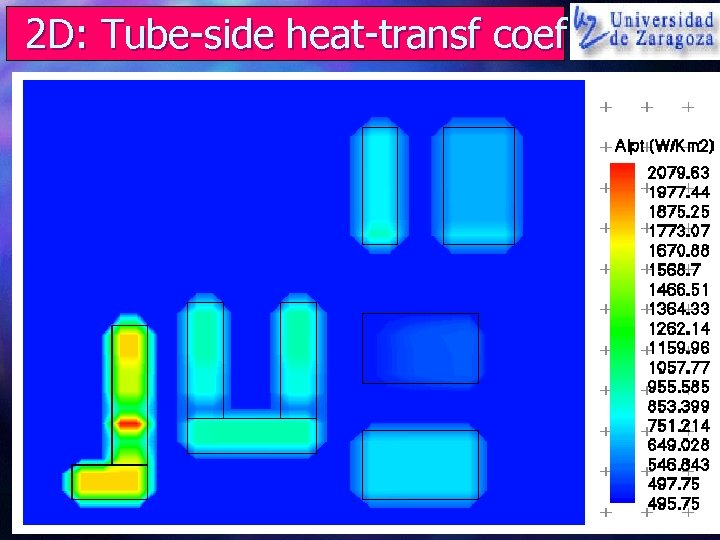2 D: Tube-side heat-transf coef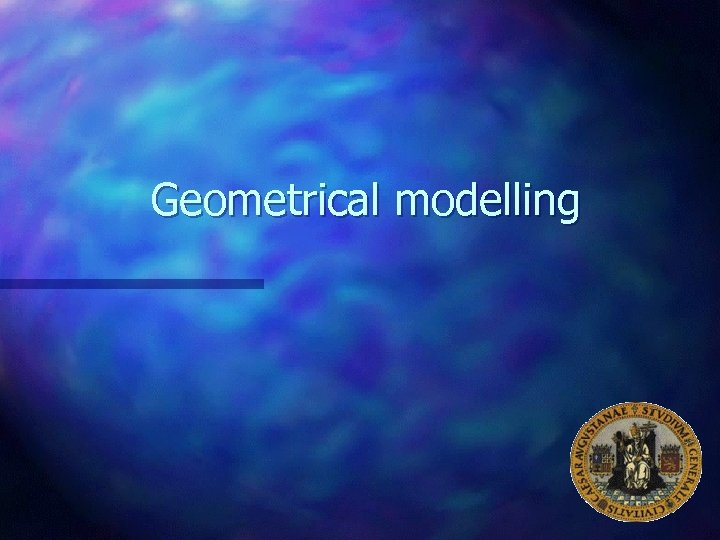Geometrical modelling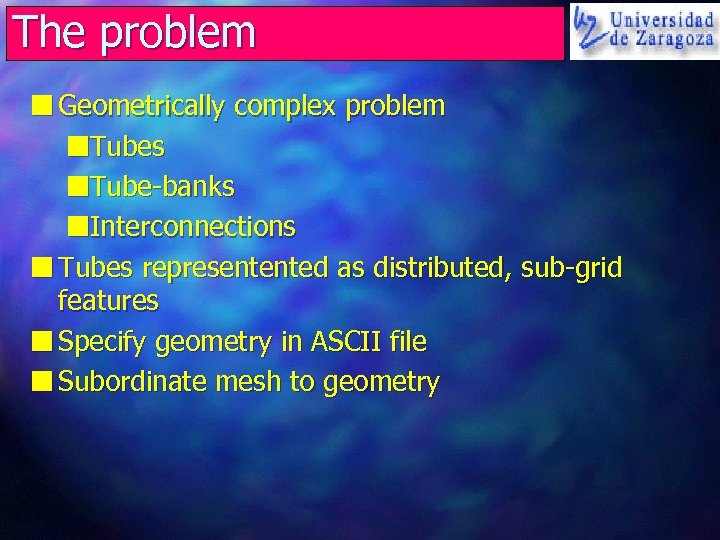The problem n Geometrically complex problem n. Tubes n. Tube-banks n. Interconnections n Tubes representented as distributed, sub-grid features n Specify geometry in ASCII file n Subordinate mesh to geometry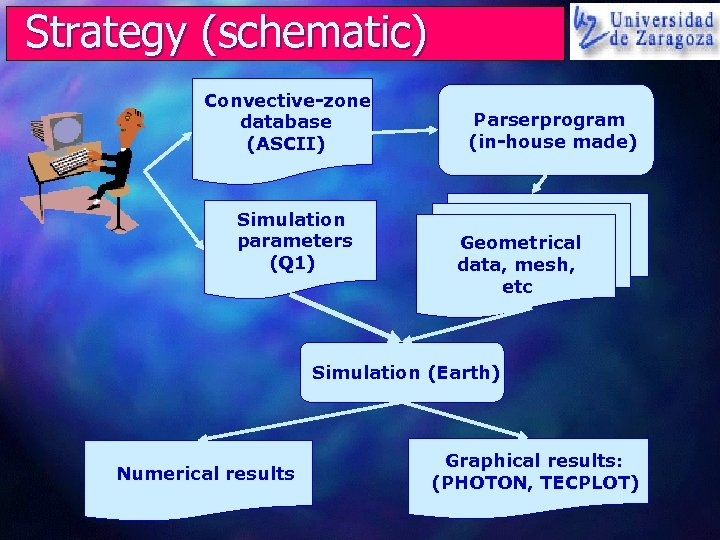Strategy (schematic) Convective-zone database (ASCII) Simulation parameters (Q 1) Parserprogram (in-house made) Geometrical data, mesh, etc Simulation (Earth) Numerical results Graphical results: (PHOTON, TECPLOT)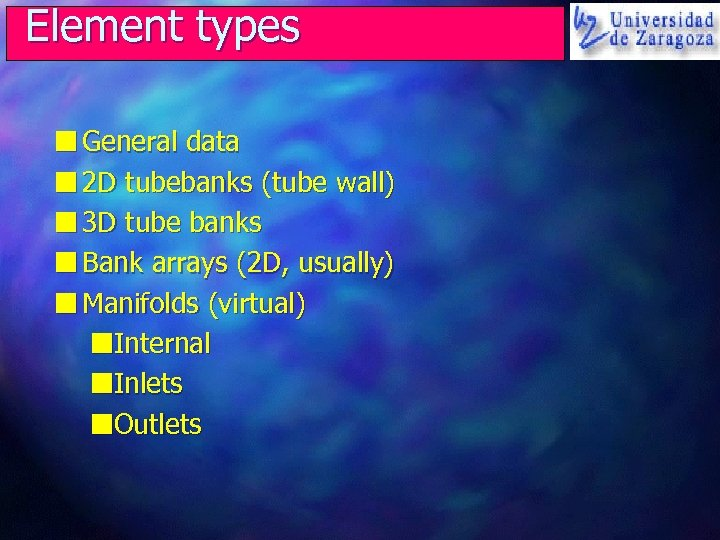Element types n General data n 2 D tubebanks (tube wall) n 3 D tube banks n Bank arrays (2 D, usually) n Manifolds (virtual) n. Internal n. Inlets n. Outlets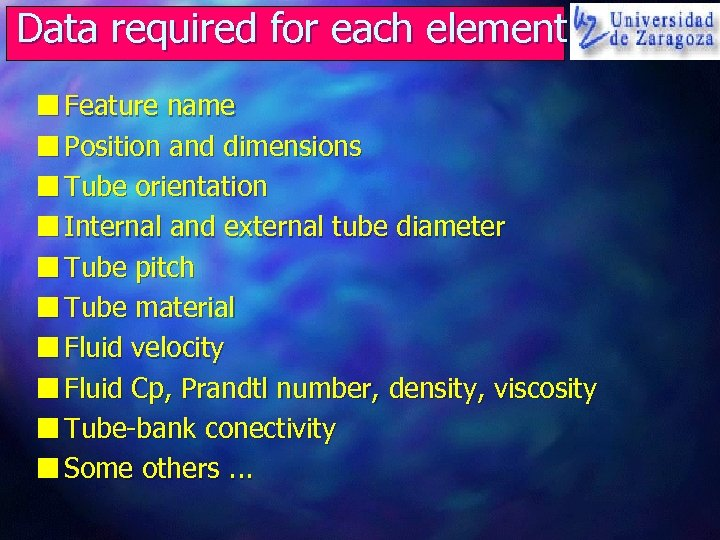Data required for each element n Feature name n Position and dimensions n Tube orientation n Internal and external tube diameter n Tube pitch n Tube material n Fluid velocity n Fluid Cp, Prandtl number, density, viscosity n Tube-bank conectivity n Some others. . .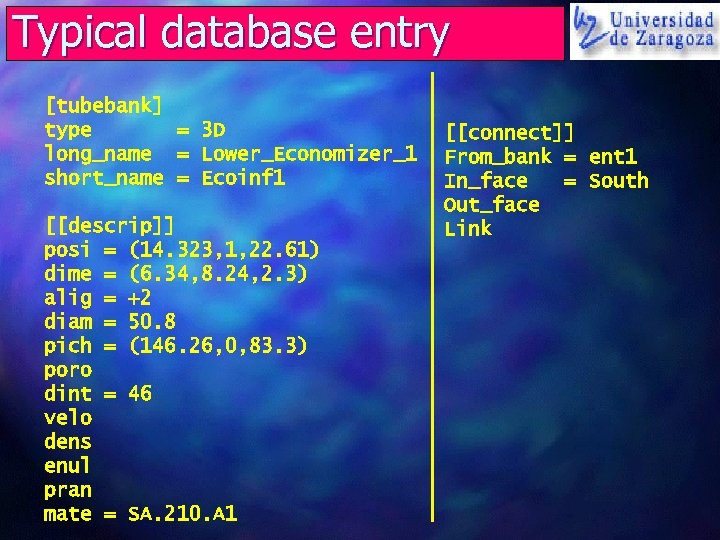Typical database entry [tubebank] type = 3 D long_name = Lower_Economizer_1 short_name = Ecoinf 1 [[descrip]] posi = (14. 323, 1, 22. 61) dime = (6. 34, 8. 24, 2. 3) alig = +2 diam = 50. 8 pich = (146. 26, 0, 83. 3) poro dint = 46 velo dens enul pran mate = SA. 210. A 1 [[connect]] From_bank = ent 1 In_face = South Out_face LinkMathematical modelling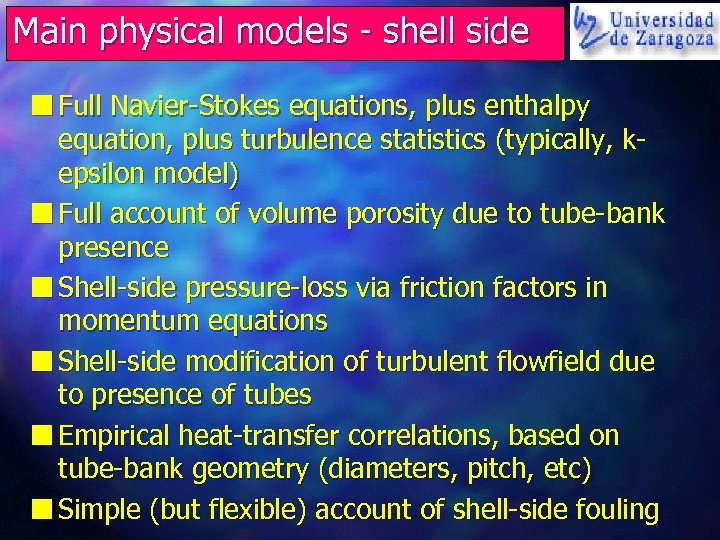Main physical models - shell side n Full Navier-Stokes equations, plus enthalpy equation, plus turbulence statistics (typically, kepsilon model) n Full account of volume porosity due to tube-bank presence n Shell-side pressure-loss via friction factors in momentum equations n Shell-side modification of turbulent flowfield due to presence of tubes n Empirical heat-transfer correlations, based on tube-bank geometry (diameters, pitch, etc) n Simple (but flexible) account of shell-side fouling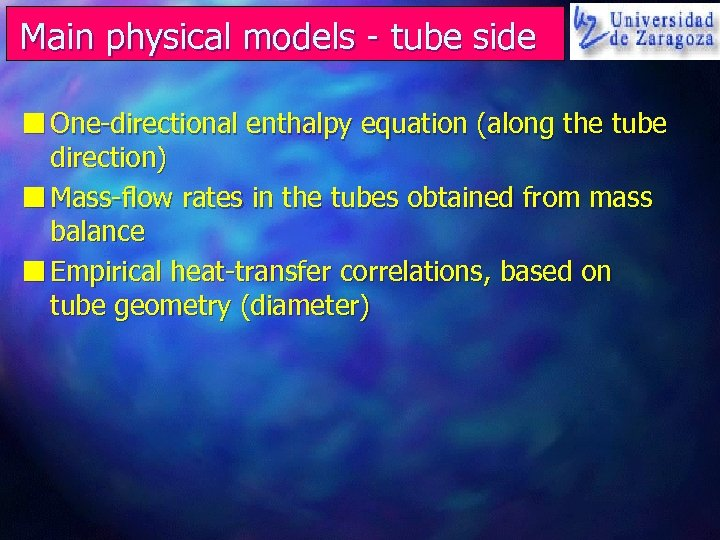Main physical models - tube side n One-directional enthalpy equation (along the tube direction) n Mass-flow rates in the tubes obtained from mass balance n Empirical heat-transfer correlations, based on tube geometry (diameter)Results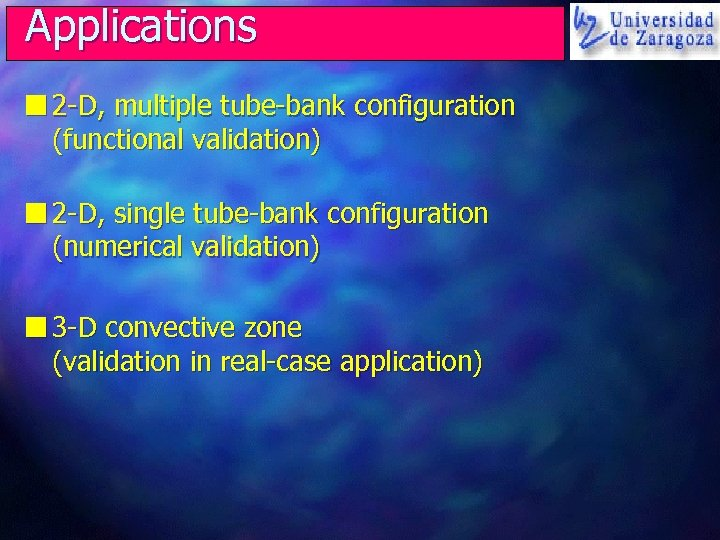Applications n 2 -D, multiple tube-bank configuration (functional validation) n 2 -D, single tube-bank configuration (numerical validation) n 3 -D convective zone (validation in real-case application)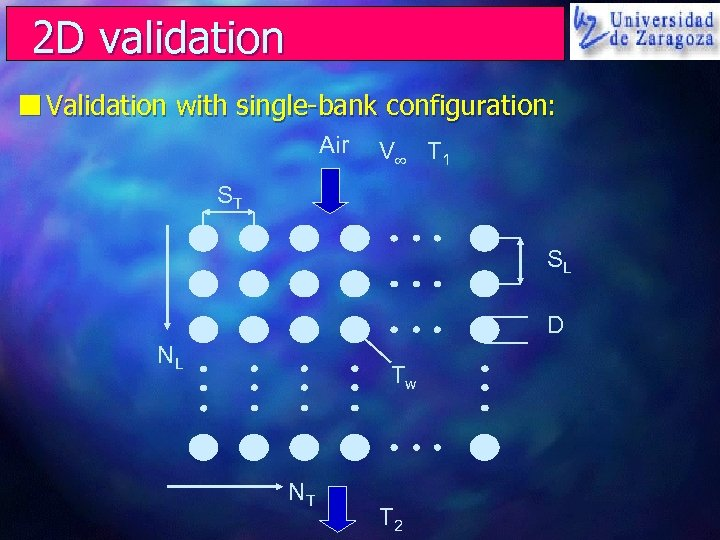2 D validation n Validation with single-bank configuration: Air V T 1 ST SL D NL Tw NT T 2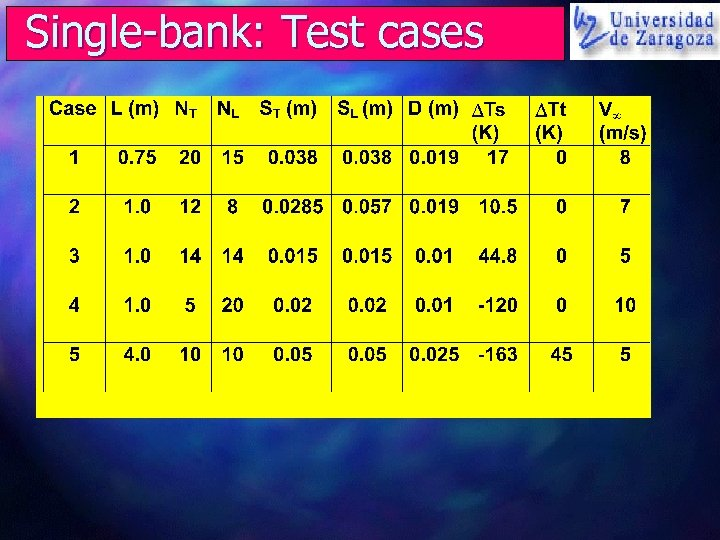Single-bank: Test casesSingle-bank: thermal results n Theory: Log Mean Temp Difference method (1 -4) and Number of Transfer Units method (5)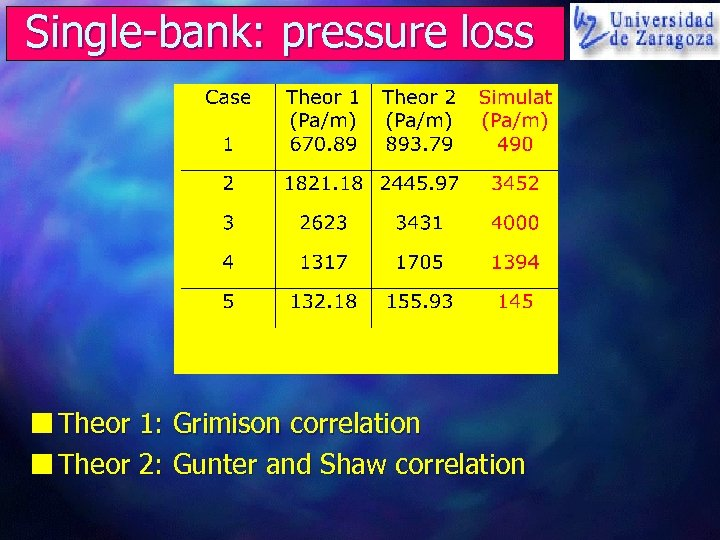Single-bank: pressure loss n Theor 1: Grimison correlation n Theor 2: Gunter and Shaw correlation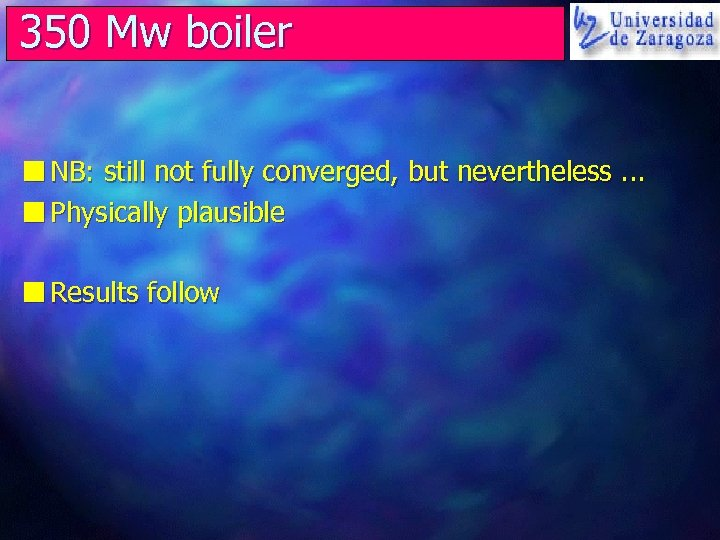350 Mw boiler n NB: still not fully converged, but nevertheless. . . n Physically plausible n Results follow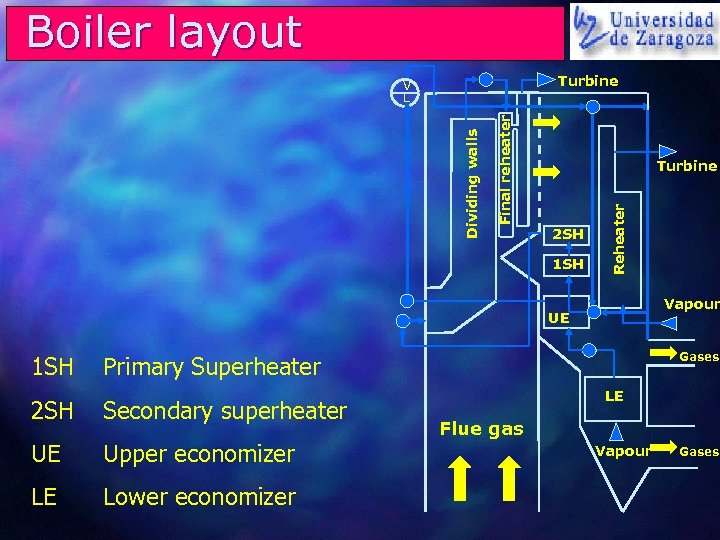Boiler layout Turbine Final reheater Turbine 2 SH 1 SH Reheater Dividing walls V L Vapour UE 1 SH Gases Primary Superheater 2 SH Secondary superheater UE Upper economizer LE Lower economizer LE Flue gas Vapour Gases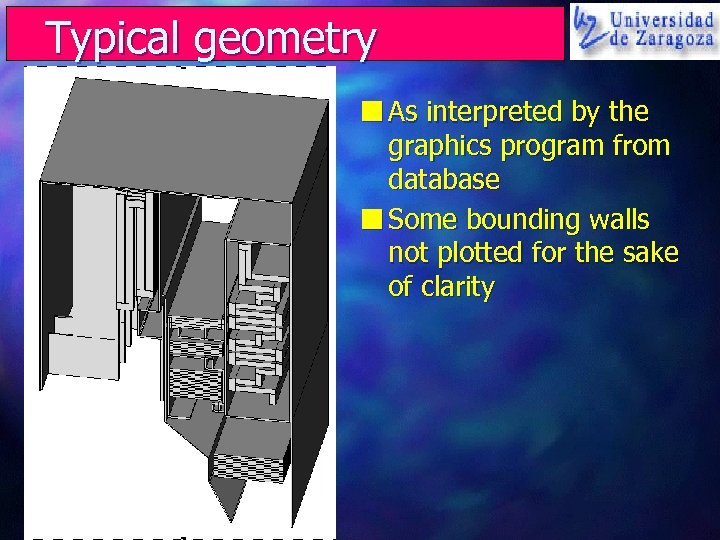Typical geometry n As interpreted by the graphics program from database n Some bounding walls not plotted for the sake of clarity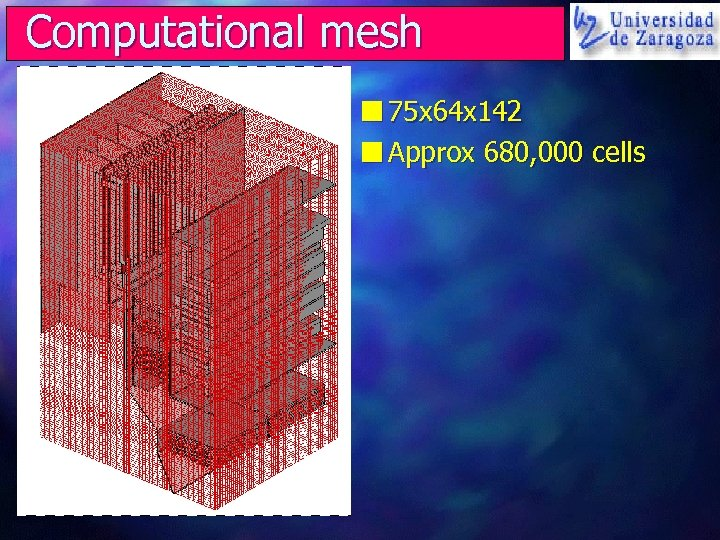Computational mesh n 75 x 64 x 142 n Approx 680, 000 cells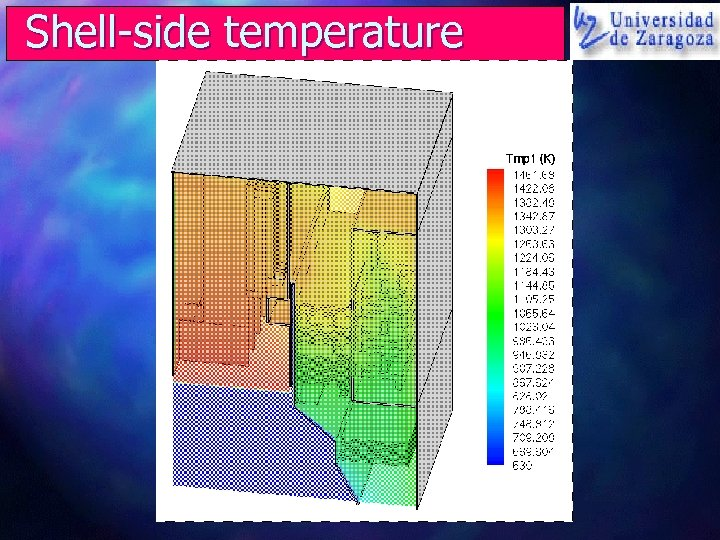Shell-side temperature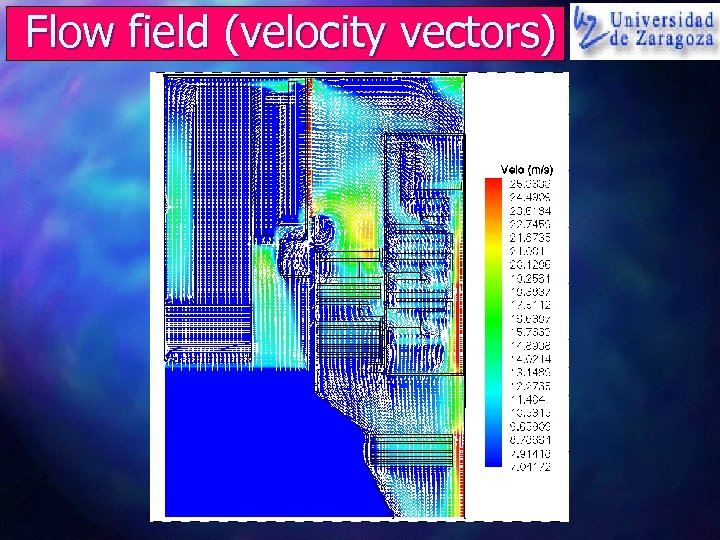Flow field (velocity vectors)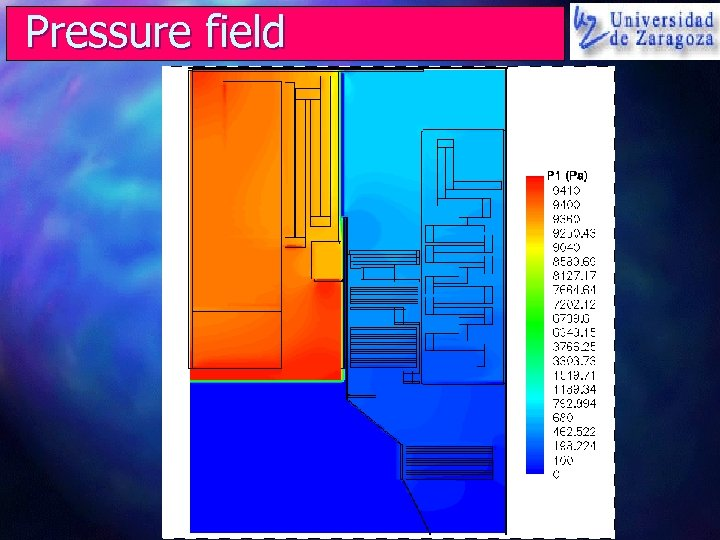Pressure field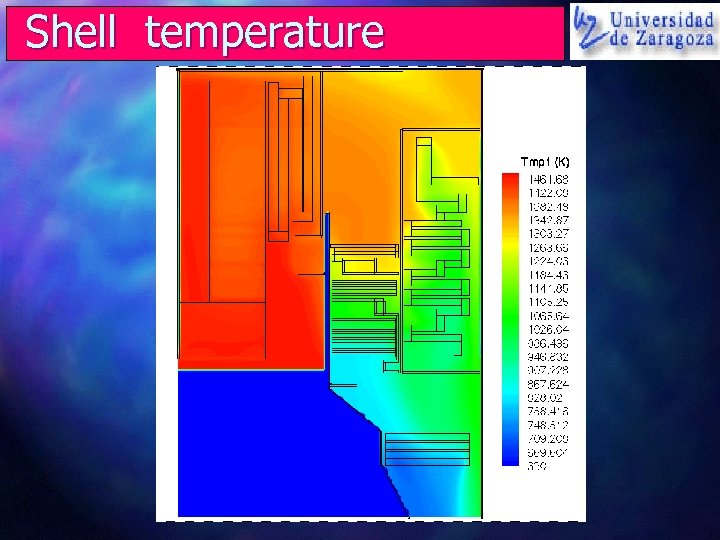Shell temperature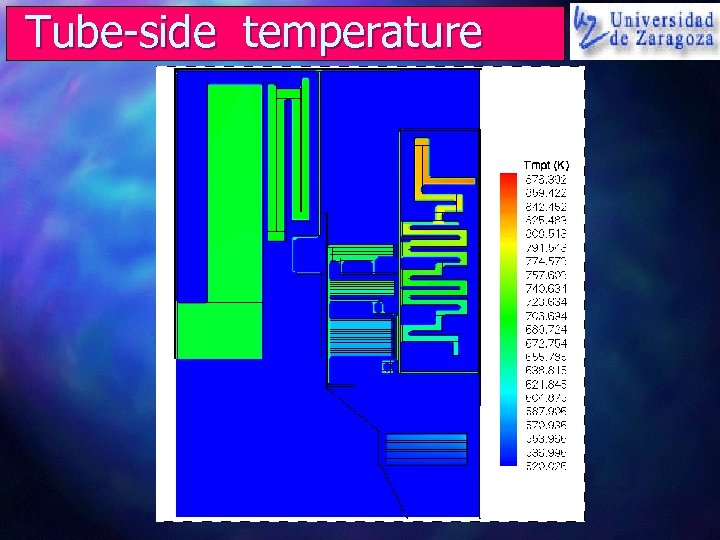Tube-side temperature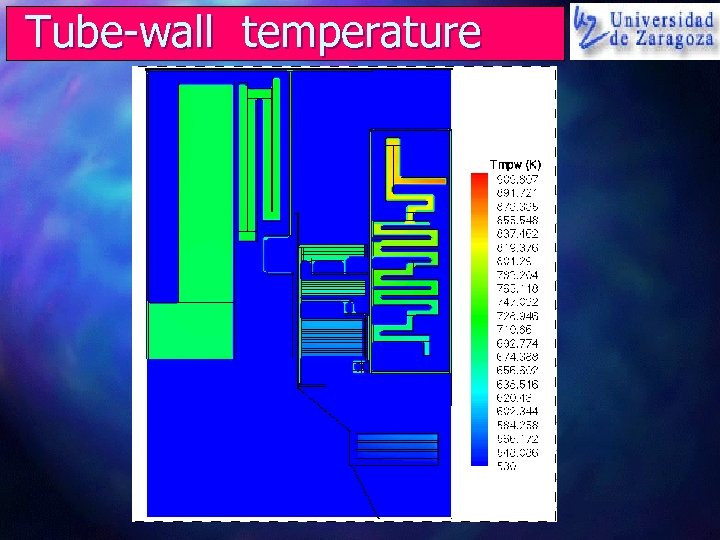Tube-wall temperature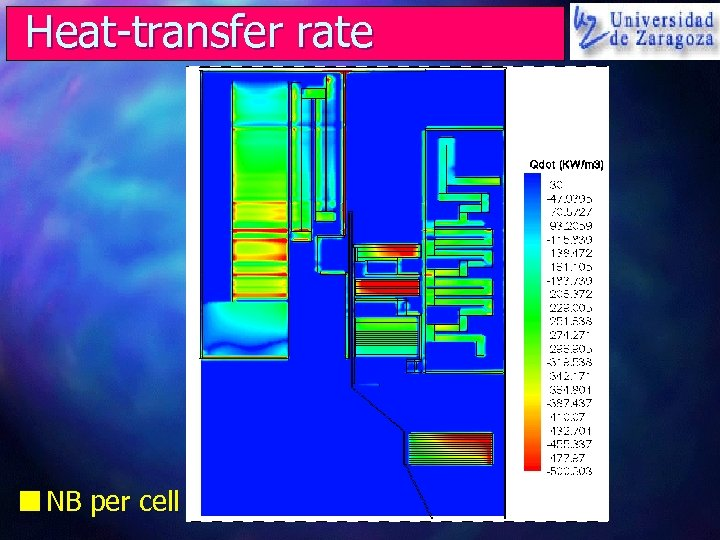Heat-transfer rate n NB per cell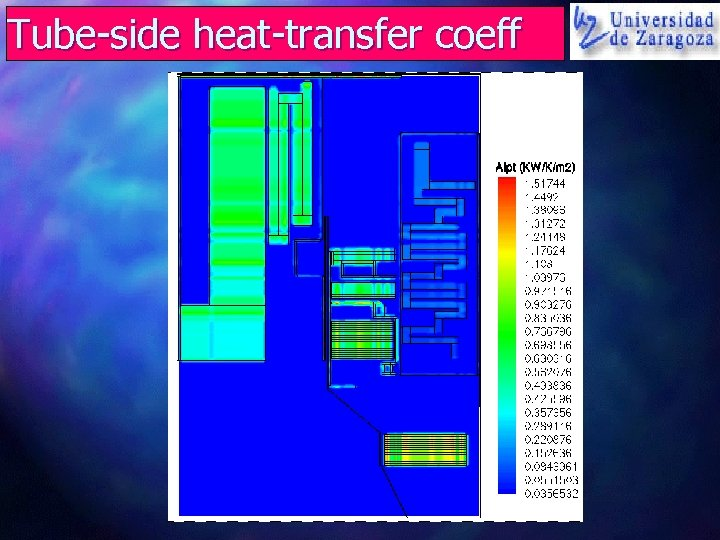Tube-side heat-transfer coeff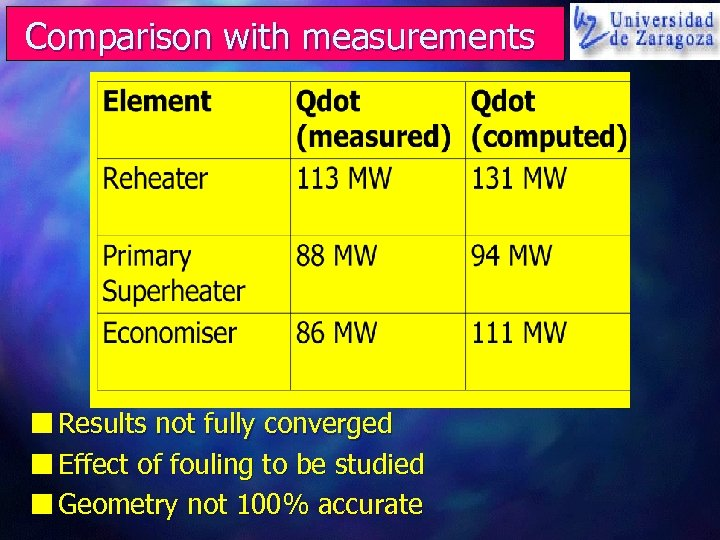Comparison with measurements n Results not fully converged n Effect of fouling to be studied n Geometry not 100% accurate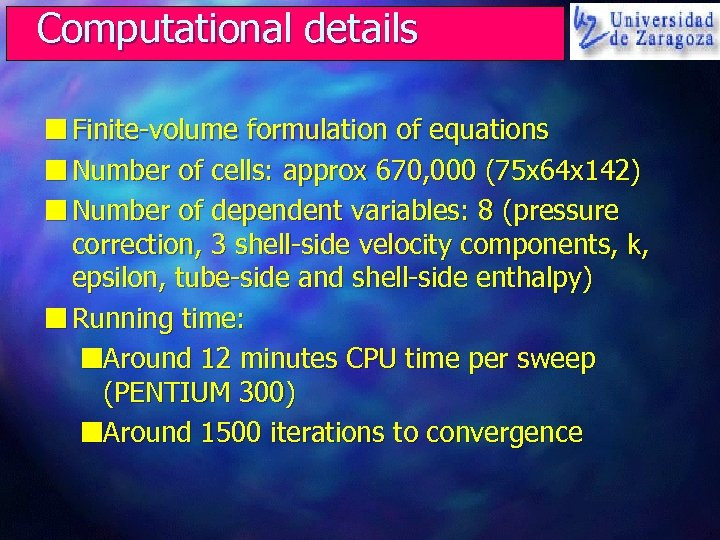Computational details n Finite-volume formulation of equations n Number of cells: approx 670, 000 (75 x 64 x 142) n Number of dependent variables: 8 (pressure correction, 3 shell-side velocity components, k, epsilon, tube-side and shell-side enthalpy) n Running time: n. Around 12 minutes CPU time per sweep (PENTIUM 300) n. Around 1500 iterations to convergence